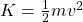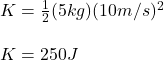## what is the kinetic energy of a 5 kg bowling ball rolling at a velocity of 10 m/s?​

Question

what is the kinetic energy of a 5 kg bowling ball rolling at a velocity of 10 m/s?​

in progress 0
1 week 2021-09-05T09:52:03+00:00 1 Answers 0 views 0

K = 250 J.

Explanation:

Hello there!

In this case, by knowing that the kinetic energy is such energy associated to the movement of an object, it is possible for us to recall the formula for its calculation in terms of mass and velocity as shown below:Thus, given the mass and velocity, it is possible to obtain the following kinetic energy:Regards!